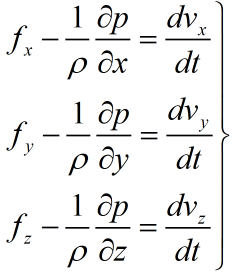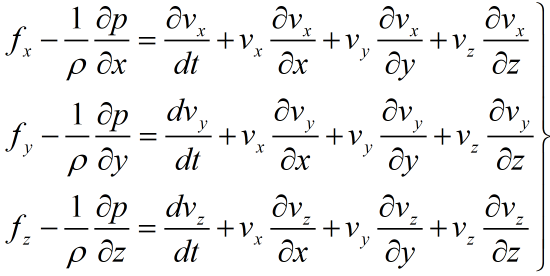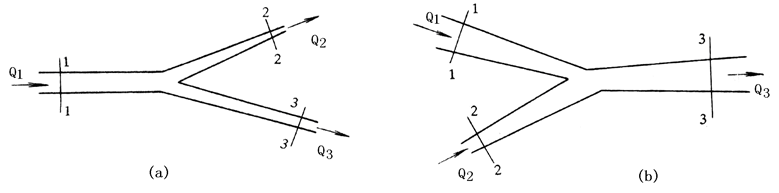• （一）理性流体运动微分方程动量守恒） （二）理想流体沿流线伯努利方程（能量守恒） （三）理想流体沿流线伯努利方程的意义 （四）理想流体总流伯努利方程 （五）实际流体总流的伯努利方程 （六）伯努利方程...
目录
（一）理性流体运动微分方程（动量守恒）
（二）理想流体沿流线伯努利方程（能量守恒）
（三）理想流体沿流线伯努利方程的意义
（四）理想流体总流伯努利方程
（五）实际流体总流的伯努利方程
（六）伯努利方程的推广
（七）存在机械能输出和输入时总的伯努利方程

（一）理性流体运动微分方程（动量守恒）
理想流体微分方程也叫做欧拉运动微分方程。是牛顿第二定律在理想流体中的应用。
表达式为：物理意义：理想流体微分方程表达了作用在单位质量流体上的力与流体运动加速度之间的关系，是流体动力学的基本方程，对于不可压缩和可压缩的流体均适用，也适用于所有的理想流体的运动。
（二）理想流体沿流线伯努利方程（能量守恒）
理想流体沿流线的伯努利方程如下所示：

适用范围
理想不可压缩流体质量力只有重力稳定流动对于有旋流动，仅适用于同一条流线；对于无旋流动，整个流场都适用。
（三）理想流体沿流线伯努利方程的意义

几何意义
z——称为位置水头；——测压管高度，速度水头；——水力高度或总水头。
压力能、动能、位能都是一种能量，他们之间可以相互转换。当流速变小时候，动能转变为压力能，压力能增加。
对于理想流体恒定流动，三项的能量之和为一常数，表示任意一个流体微元运动过程中的位能、压力能和动能的总和保持不变。所以说理想流体，伯努利方程又是流体力学中的能量守恒定量。
动能修正系数
动能修正系数是过流断面流体流动的真实速度所表示的动能与过流断面平均速度所表示的动能之比，用字母α表示。
即：
上式说明过断流面平均速度计算得到的动能要小于用过断流面真实速度计算所得的动能。是因为断面上速度分布不均匀所引起的，不均匀性越大，α值越大。在实际中，由于流速水头本身所占的比例较小，所以一般取α=1。
缓变流及其特征
缓变流：值流线之间的夹角比较小，流线曲率半径比较大，流线几乎是一些平行直线的流动。
在缓变流中，流体运动的直线加速度和离心加速度都很小，可以忽略由于速度的变化或者方向的变化所产生的惯性力。
缓变过流断面：如果在流束的某一过流面上的流动为缓变流动，则称此断面为缓变过流断面。
缓变过流具有以下两个特征
缓变流动中，质量力只有重力。在同一缓变过流断面上，任何点上的静压水头都相等。
（四）理想流体总流伯努利方程

适用条件
理想不可压缩流体在重力场下的稳定缓变流动。
（五）实际流体总流的伯努利方程
实际流体总流的伯努利方程式：

适用条件
理想不可压缩流体。作用在流体上的质量力只有重力。稳定流动。沿流程流量保持不变。所取的过流断面必须是缓变流断面。
伯努利方程的使用注意事项
与总流的连续性方程式联合使用。在选取过流断面时，一个过流断面应选在待求未知量所在的断面上，另一个过流断面需要选在已知量较多的断面上，且尽可能使两个断面只包含一个未知数；为了方便，基准面通常选在过流断面的最低的一个断面上，在同一个问题中，必须使用同一个基准面。选择的计算点，位置高度z和压力p必须在同一点上。压力可以用绝对压力，也可以使用相对压力，但是两个断面上所用的压力标准必须一致。所选择的过流断面必须满足缓变流动条件，但在两个缓变过流断面之间的流动，可以是缓变流动也可以是急变流动。方程中动能修正系数α≈1
（六）伯努利方程的推广
流体在流动过程中有分流和汇流。分流过程中有，断面1和2、断面1和3之间的伯努利方程为：

在第一个式子和第二个式子两边分别乘以再相加，得到总能量守恒的伯努利方程：

对于汇流情况，同理列出1、3和2、3的伯努利方程，得到总能量守恒的伯努利方程：

（七）存在机械能输出和输入时总的伯努利方程
沿着总流两过流断面间装有水泵、风机和水轮机等装置，流体流经水泵或者风机时候获得能量，流经水轮机时将失去能量。设流体获得或失去能量头为H，则总流伯努利方程为：

H前的正号表示获得的能量，负号表示失去的能量。
以上内容大多来自网络。
展开全文• 2.66为守恒形式的动量方程。 教材截图来自 何雅玲, 王勇, 李庆. 格子Boltzmann方法的理论及应用[M]. 科学出版社, 2009. Material derivative来自 Timm Krüger, Kusumaatmaja H , Kuzmin A , et al. The ...2.66为守恒形式的动量方程。
教材截图来自 何雅玲, 王勇, 李庆. 格子Boltzmann方法的理论及应用[M]. 科学出版社, 2009.Material derivative来自 Timm Krüger, Kusumaatmaja H , Kuzmin A , et al. The Lattice Boltzmann method : principles and practice[M]. 2017.展开全文流体力学
• 麦克斯韦方程组⎧⎩⎨⎪⎪⎪⎪⎪⎪⎪⎪⎪⎪⎪⎪∇⋅E=ρε0∇×E=−∂B∂t∇⋅B=0∇×B=μ0j\begin{cases} \nabla\cdot\mathbf E=\dfrac{\rho}{\varepsilon_0}\\ \nabla\times\mathbf E=-\dfrac{\partial B}{\...
麦克斯韦方程组

⎧⎩⎨⎪⎪⎪⎪⎪⎪⎪⎪⎪⎪⎪⎪∇⋅E=ρε0∇×E=−∂B∂t∇⋅B=0∇×B=μ0j

\begin{cases}
\nabla\cdot\mathbf E=\dfrac{\rho}{\varepsilon_0}\\
\nabla\times\mathbf E=-\dfrac{\partial B}{\partial t}\\
\nabla\cdot\mathbf B=0\\
\nabla\times\mathbf B=\mu_0\mathbf j
\end{cases}

∇⋅(∇×E)=∇⋅(−∂B∂t)=−∂(∇⋅B)∂t=0

\nabla\cdot(\nabla\times\mathbf E)=\nabla\cdot(-\dfrac{\partial B}{\partial t})=-\dfrac{\partial (\nabla\cdot \mathbf B)}{\partial t}=0

这说明前两个方程是合理、自洽的.但是

∇⋅(∇×B)=∇⋅(μ0j)=μ0(−∂ρ∂t)

\nabla\cdot(\nabla\times\mathbf B)=\nabla\cdot(\mu_0\mathbf j)=\mu_0(-\dfrac{\partial\rho}{\partial t})

结果并不总是等于

0
$0$,为此，Maxwell引入位移电流 jd$\mathbf j_d$，即

∇×B=μ0(j+jd)

\nabla\times\mathbf B=\mu_0(\mathbf{ j+j}_d)
由此

∇⋅(∇×B)=∇⋅[μ0(j+jd)]=μ0[∇⋅jd−∂ρ∂t]=μ0[∇⋅jd−ε0∂(∇⋅E)∂t]=0⇒jd≡ε0∂E∂t

\nabla\cdot(\nabla\times\mathbf B)=\nabla\cdot[\mu_0(\mathbf{ j+j}_d)]=\mu_0[\nabla\cdot \mathbf j_d-\dfrac{\partial\rho}{\partial t}]=\mu_0[\nabla\cdot \mathbf j_d-\varepsilon_0\dfrac{\partial(\nabla\cdot\mathbf E)}{\partial t}]=0\\\Rightarrow\mathbf j_d\equiv\varepsilon_0\dfrac{\partial \mathbf E}{\partial t}

所以方程组修改为

⎧⎩⎨⎪⎪⎪⎪⎪⎪⎪⎪⎪⎪⎪⎪⎪⎪⎪⎪∇⋅E=ρε0∇×E=−∂B∂t∇⋅B=0∇×B=μ0j+ε0μ0∂E∂t

\begin{cases}
\nabla\cdot\mathbf E=\dfrac{\rho}{\varepsilon_0}\\
\nabla\times\mathbf E=-\dfrac{\partial B}{\partial t}\\
\nabla\cdot\mathbf B=0\\
\nabla\times\mathbf B=\mu_0\mathbf j+\varepsilon_0\mu_0\dfrac{\partial \mathbf E}{\partial t}
\end{cases}

洛伦兹力方程
宏观

F=qE+qv×B

\mathbf F=q\mathbf E+q\mathbf{v\times B}
微观

f=ρE+j×B

\mathbf f=\rho\mathbf E+\mathbf j\times \mathbf B
Maxwell方程组和洛伦兹力方程一起构成了经典电动力学的基础。

能量守恒
能量守恒的目标形式是

∭Wdτ−ddt∭ωdτ−∮S⋅dσ=∭Wdτ−ddt∭ωdτ−∭∇⋅Sdτ⇒W=−dωdt−∇⋅S

\iiint W\mathrm d\tau-\dfrac{\mathrm d}{\mathrm dt}\iiint\omega\mathrm d\tau-\oint\mathbf S\cdot\mathrm d\sigma\\=\iiint W\mathrm d\tau-\dfrac{\mathrm d}{\mathrm dt}\iiint\omega\mathrm d\tau-\iiint\nabla\cdot\mathbf S\mathrm d\tau\\\Rightarrow W=-\dfrac{\mathrm d\omega}{\mathrm dt}-\nabla\cdot\mathbf S

其中

W
$W$是一定空间V$V$内在合体能量增加率，

ω
$\omega$是电磁场能量密度，

S
$\mathbf S$是能流密度.

W=E⋅j=E⋅(1μ0∇×B−ε0∂E∂t)=1μ0E⋅(∇×B)−ε0E⋅∂E∂t=1μ0[−∇⋅(E×B)+B⋅(∇×E)]−ε0E⋅∂E∂t=−1μ0∇⋅(E×B)+1μ0B⋅(∇×E)−ε0E⋅∂E∂t=−1μ0∇⋅(E×B)+1μ0B⋅(−∂B∂t)−ε0E⋅∂E∂t=−∇⋅[1μ0(E×B)]−12∂∂t[1μ0B2+ε0E2]

\begin{array}
&W=\mathbf{E\cdot j}
\\=\mathbf E\cdot(\dfrac1{\mu_0}\nabla\times \mathbf B-\varepsilon_0\dfrac{\partial\mathbf E}{\partial t})
\\=\dfrac1{\mu_0}\mathbf E\cdot(\nabla\times \mathbf B)-\varepsilon_0\mathbf E\cdot\dfrac{\partial\mathbf E}{\partial t}
\\=\dfrac1{\mu_0}[-\nabla\cdot(\mathbf E\times \mathbf B)+\mathbf B\cdot(\nabla\times \mathbf E)]-\varepsilon_0\mathbf E\cdot\dfrac{\partial\mathbf E}{\partial t}
\\=-\dfrac1{\mu_0}\nabla\cdot(\mathbf E\times \mathbf B)+\dfrac1{\mu_0}\mathbf B\cdot(\nabla\times \mathbf E)-\varepsilon_0\mathbf E\cdot\dfrac{\partial\mathbf E}{\partial t}
\\=-\dfrac1{\mu_0}\nabla\cdot(\mathbf E\times \mathbf B)+\dfrac1{\mu_0}\mathbf B\cdot(-\dfrac{\partial\mathbf B}{\partial t})-\varepsilon_0\mathbf E\cdot\dfrac{\partial\mathbf E}{\partial t}
\\=-\nabla\cdot\left[\dfrac1{\mu_0}(\mathbf E\times \mathbf B)\right]-\dfrac12\dfrac{\partial}{\partial t}\left[\dfrac1{\mu_0} B^2+\varepsilon_0 E^2\right]
\end{array}

比较一下理想形式，可以得到

S=1μ0(E×B)ω=12(1μ0B2+ε0E2)

\mathbf S=\dfrac1{\mu_0}(\mathbf E\times \mathbf B)\\\omega=\dfrac12\left(\dfrac1{\mu_0} B^2+\varepsilon_0 E^2\right)

动量守恒
动量守恒的目标形式是

∭fdτ−ddt∭gdτ−∮T⃗ ⋅dσ=∭fdτ−ddt∭gdτ−∭∇⋅T⃗ dτ⇒f=−dgdt−∇⋅T⃗

\iiint \mathbf f\mathrm d\tau-\dfrac{\mathrm d}{\mathrm dt}\iiint\mathbf g\mathrm d\tau-\oint\vec{\mathbf T}\cdot\mathrm d\sigma\\=\iiint \mathbf f\mathrm d\tau-\dfrac{\mathrm d}{\mathrm dt}\iiint\mathbf g\mathrm d\tau-\iiint\nabla\cdot\vec{\mathbf T}\mathrm d\tau\\\Rightarrow \mathbf f=-\dfrac{\mathrm d\mathbf g}{\mathrm dt}-\nabla\cdot\vec{\mathbf T}

其中

f
$\mathbf f$是一定空间

V
$V$内在合体动能增加率，g$\mathbf g$是电磁场动量密度，

T⃗
$\vec{\mathbf T}$是动量流密度.

f=ρE+j×B=ε0(∇⋅E)E+(1μ0∇×B−ε0∂E∂t)×B=ε0(∇⋅E)E+1μ0(∇×B)×B−ε0∂E∂t×B

\begin{array}
&\mathbf f=\rho\mathbf E+\mathbf j\times \mathbf B
\\=\varepsilon_0(\nabla\cdot \mathbf E)\mathbf E+(\dfrac1{\mu_0}\nabla\times \mathbf B-\varepsilon_0\dfrac{\partial\mathbf E}{\partial t})\times \mathbf B
\\=\varepsilon_0(\nabla\cdot \mathbf E)\mathbf E+\dfrac1{\mu_0}(\nabla\times \mathbf B)\times \mathbf B-\varepsilon_0\dfrac{\partial\mathbf E}{\partial t}\times \mathbf B
\end{array}
利用Maxwell方程组的另外两个方程

0=1μ0(∇⋅B)B+ε0(∇×E)×E+ε0∂B∂t×E

0=\dfrac1\mu_0(\nabla\cdot \mathbf B)\mathbf B+\varepsilon_0(\nabla\times \mathbf E)\times \mathbf E+\varepsilon_0\dfrac{\partial\mathbf B}{\partial t}\times \mathbf E

两式相加，得

f=ε0[(∇⋅E)E+(∇×E)×E]+1μ0[(∇⋅B)B+(∇×B)×B]−ε0[∂E∂t×B−∂B∂t×E]

\begin{array}
&\mathbf f=\varepsilon_0[(\nabla\cdot \mathbf E)\mathbf E+(\nabla\times \mathbf E)\times \mathbf E]+\dfrac1\mu_0[(\nabla\cdot \mathbf B)\mathbf B+(\nabla\times \mathbf B)\times \mathbf B]-\varepsilon_0\left[\dfrac{\partial\mathbf E}{\partial t}\times \mathbf B-\dfrac{\partial\mathbf B}{\partial t}\times \mathbf E\right]
\end{array}
因为

∇⋅(EE)=(E⋅∇)E+(∇⋅E)E(∇×E)×E=(E⋅∇)E−12∇E2

\nabla\cdot(\mathbf E\mathbf E)=(\mathbf E\cdot \nabla)\mathbf E+(\nabla\cdot \mathbf E)\mathbf E\\(\nabla\times \mathbf E)\times \mathbf E=(\mathbf E\cdot\nabla)\mathbf E-\dfrac12\nabla E^2
所以

(∇⋅E)E+(∇×E)×E=∇⋅(EE)−12∇E2=∇⋅(EE)−12∇⋅(E2I⃗ )=∇⋅(EE−12E2I⃗ )

(\nabla\cdot \mathbf E)\mathbf E+(\nabla\times \mathbf E)\times \mathbf E=\nabla\cdot(\mathbf E\mathbf E)-\dfrac12\nabla E^2\\=\nabla\cdot(\mathbf E\mathbf E)-\dfrac12\nabla\cdot( E^2\vec{\mathbf I})\\=\nabla\cdot(\mathbf E\mathbf E-\dfrac12 E^2\vec{\mathbf I})
同理

(∇⋅B)B+(∇×B)×B=∇⋅(BB−12B2I⃗ )

(\nabla\cdot \mathbf B)\mathbf B+(\nabla\times \mathbf B)\times \mathbf B=\nabla\cdot(\mathbf B\mathbf B-\dfrac12 B^2\vec{\mathbf I})
又有

∂∂t(E×B)=∂E∂t×B+E×∂B∂t=∂E∂t×B−∂B∂t×E

\dfrac{\partial}{\partial t}(\mathbf E\times \mathbf B)=\dfrac{\partial\mathbf E}{\partial t}\times \mathbf B+\mathbf E\times \dfrac{\partial\mathbf B}{\partial t}\\=\dfrac{\partial\mathbf E}{\partial t}\times \mathbf B- \dfrac{\partial\mathbf B}{\partial t}\times\mathbf E

所以得到

f=−ε0∂∂t(E×B)−∇⋅(12(ε0E2I⃗ +1μ0B2I⃗ )−ε0EE−1μ0BB)

\begin{array}
&\mathbf f=-\varepsilon_0\dfrac{\partial}{\partial t}(\mathbf E\times \mathbf B)-\nabla\cdot\left(\dfrac12( \varepsilon_0E^2\vec{\mathbf I}+\dfrac1{\mu_0}B^2\vec{\mathbf I})-\varepsilon_0\mathbf E\mathbf E-\dfrac1{\mu_0}\mathbf B\mathbf B\right)
\end{array}
比较一下理想形式，可以得到

g=ε0E×BT⃗ =12(ε0E2I⃗ +1μ0B2I⃗ )−ε0EE−1μ0BB

\mathbf {g}={\varepsilon_0 }\mathbf E\times \mathbf B\\\vec{\mathbf T}=\dfrac12( \varepsilon_0E^2\vec{\mathbf I}+\dfrac1{\mu_0}B^2\vec{\mathbf I})-\varepsilon_0\mathbf E\mathbf E-\dfrac1{\mu_0}\mathbf B\mathbf B

g=ε0μ0S=Sc2

\mathbf g=\varepsilon_0\mu_0\mathbf S=\dfrac{\mathbf S}{c^2}

本篇主要参考俞允强《电动力学简明教程》

展开全文• 动能公式：   动量公式:   动量守恒：   能量守恒:    根据这些规律可以得到下列方程组:     ...解该方程组，得到下面的公式: ...基本的动量守恒演示：
 动能公式：

动量公式:

动量守恒：

能量守恒:

根据这些规律可以得到下列方程组:

解该方程组，得到下面的公式:

把这二个公式相减，可以得到:

即：

我们也经常利用这个公式简化运算

基本的动量守恒演示：
先给ball类添加一个质量"属性"

View Code

一维单轴刚体碰撞测试：

View Code

二维坐标上的刚体碰撞：先来看这张图，红球a以Va速度运动，蓝球b以Vb速度运动，二球的连线正好与x轴平行(即：水平对心碰撞)，碰撞的过程可以理解为二球水平速度分量Vax,Vbx应用运量守恒与能力守恒的结果(y轴方向的速度不受影响！)
但很多情况下，二球的连线并非总是与坐标轴平行，比如下面这样：思路：仍然利用坐标旋转，先将二个球反向旋转到连线水平位置，然后按常规方式处理，完事后再旋转回来。

View Code

粘连问题：
反复运行上面这段动画，偶尔可能会发现二个球最终粘在一起，无法分开了，造成这种原因的情况很多，下面的示意图分析了可能的形成原因之一解决思路：找出重叠部分，然后把二个小球同时反向移动适当距离，让二个球分开即可先来一段测试代码：验证一下是否有效

View Code

水平拖动小球故意让它们重叠，然后点击“分开”按钮测试一下，ok，管用了！
再回过头来解决运量守恒中的粘连问题:
只要把EnterFrameHandler中的

?

1

2

3

4

5

//相对位置处理 

xA+=vxAFinal; 

xB+=vxBFinal; 

换成:

?

1

2

3

4

5

6

7

8

9

10

11

12

13

14

15

16

17

18

19

20

21

22

//相对位置处理(同时要防止粘连)

//xA+=vxAFinal;

//xB+=vxBFinal;

var
sumRadius = ballA.radius + ballB.radius;

var
overlap:
Number
=sumRadius-Math.abs(xA-xB);
//计算重叠部分

//trace(overlap);

    

//计算每个球所占重叠部分中的比例

var
aRadio:
Number
= ballA.radius/sumRadius;

var
bRadio:
Number
= ballB.radius/sumRadius;

    

//分离判断

if
(overlap>
0
){

    
if
(xA>xB){

        
xA += overlap*aRadio;

        
xB -= overlap*bRadio;

    
}

    
else
{

        
xA -= overlap*aRadio;

        
xB += overlap*bRadio;

    
}

}

最后老规矩：来一个群魔乱舞，把一堆球放在一块儿乱撞

View Code

注：这段代码做了优化，把一些公用的部分提取出来封装成function了，同时对于粘连问题的解决，采用了更一种算法

后记：弄懂了本文中的这些玩意儿有啥用呢？让我想想，或许...公司需要开发一款桌面台球游戏时，这东西就能派上用场吧.
展开全文测试 function 算法 优化
• 动量守恒： 能量守恒: 根据这些规律可以得到下列方程组: 解该方程组，得到下面的公式: 把这二个公式相减，可以得到: 即： 我们也经常利用这个公式简化运算 基本的动量守恒演示： 先给ball类...Flash Flex 算法
• HDU 5066 Harry And Physical Teacher(物理 动量守恒 动能守恒)——BestCoder Round #14acm
• package { import flash.display.Sprite; public class Ball extends Sprite { public var radius:Number; public var color:uint; public var vx:Number=0; public
• 确实，虽然这个世界瞬息万变，沧海桑田，中国更是日新月异，但总有些东西是守恒的，目前人们发现的有质量守恒、电荷守恒、能量守恒、动量守恒等，还有别的在某些条件下才成立的守恒。在经典力学里，动量守恒是牛三...
• 方法取自安德森《计算流体力学基础》 连续性方程 固定流体微元内质量变化率=流体从笛卡尔坐标三个方向流出量 因此可得： ...动量方程（纳维-斯托克斯方程） 根据牛顿第二定律可以得出：F=ma；
• 试证明: 利用连续性方程及动量方程, 能量守恒方程 (2. 15) 可化为 (2. 21) 的形式. 证明: 注意到 $$\beex \bea &\quad\cfrac{\p}{\p t}\sex{\cfrac{1}{2}\rho u^2} +\Div\sez{\cfrac{1}{2}\rho u^2{\bf u}-{\... • 刚体的动量方程可由 刚体的动量方程两端叉乘r得到 水平射流问题 假设入口面为3截面出口面为1 2截面 出口速度v1 v2 是未知的 为了研究方便 ** ** 建立xoy坐标系 其中x 与挡板垂直y与挡板相平行 假设流体作用在...1024程序员节 • 在阅读复习指导时，最好将课本同时打开，对照进行相关的推导练习。本指导是依据马文蔚、周雨青编写的《物理学教程》第三版（高等教育出版社出版）撰写的。 书本的公式推导过程基本上很清晰。本指导的主要内容是... • 1. 设真空中运动的带电体的总机械能为 U_m, 则$$\beex \bea \cfrac{\rd U_m}{\rd t} &=\int_\Omega {\bf f}\cdot {\bf v} \rd V\quad\sex{\mbox{功能关系}}\\ &=\int_\...2. 上式即代表电磁能量守恒与转化定律.
• 不同文献中动量方程的表达形式也多种多样，现总结如下： 守恒形式： ∂(ρU)∂t=−∇⋅(ρUU)−∇⋅τ−∇p+ρg\frac{\partial( \rho \mathbf U)}{\partial t}=-\nabla \cdot {(\rho\mathbf U \mathbf U ) }-\...openfoam
• http://www.cfd-china.com/topic/389/对控制方程有些疑惑-路过大神给解解惑吧/7
• 1. 场电荷系统能动量转化和守恒定律的一般形式 只考虑两个电流元组成的体系，它们的动量是不守恒的（见【真空中的基本实验定律】），即它们组成的系统不是封闭的，所以需要考虑电磁场所携带的能量与动量。场是弥漫...
• ## 流体动力学控制方程（详细推导）

万次阅读 多人点赞 2018-07-06 18:16:00
流体流动要受到物理守恒定律的支配，基本的守恒定律包括：质量守恒定律、动量守恒定律、能量守恒定律。控制方程（governing equations）是这些守恒定律的数学描述。本篇文章将对三大流体力学方程进行推导，以加深对...流体力学
• 在这3个流形上的渐近爱因斯坦方程和正则条件表明，过去零无穷大的相关Weyl张量分量与未来零无穷大的相关的Weyl张量是对映匹配的。 接近空间无穷大的完全无通量超翻译的子代数在过去和将来的零无穷大的BMS超翻译之间...
• 现在的工作主要涉及到三大守恒定律中的质量守恒以及动量守恒方程，外加一个传输扩散方程。 目前数学模型已经建立完毕，主要是如何编程求解，现有的方法比较多，有限差分法、有限体积法是最常用的，但是我的基础在...
• 为研究非饱和岩体对温度场-渗流场-变形场三场耦合作用的响应，采用等效连续介质模型，根据质量守恒方程、线动量平衡方程和能量守恒方程以及相应的物性方程（状态方程和本构方程）推导了非饱和岩体三场耦合控制方程，...
• 此模型方程式满足宏观的质量、总动量和总能量守恒方程式，满足Ｈ定理，并且在分子流粘性流两个极限情况下给出与玻氏方程式相同的解答。因此可以近似地用此模型方程式代替玻氏方程式讨论介于分子流粘性流之间的...
• 我们用数字方法计算DSG模型的第一组非平凡独立的电荷（超出能量和动量）：伪势方法中的两个三阶守恒电荷两个五阶渐近守恒电荷，以及前四个异常 分别收费的新塔。 对于Bazeia等人，我们考虑扭结，扭结抗扭结构...
• 忙碌了一个学期终于放暑假了，小白心情很愉快。然而想起CFD教材上的那些点缀着各种让人眼花缭乱符号的数学公式，整个人就不好了。不过这些事情小白也...律，最常见的流动控制方程包括质量守恒方程、动量守恒方程...
• 我们考虑一类高阶导数3d向量场模型，其中场方程算子是Chern–Simons算子的多项式。 对于这种类型的n阶理论，我们... 该族包括无界的规范能量动量张量，而有界的守恒张量为系统中的某些参数组合提供经典的系统稳定性。
• 勒让德变换正则方程 设有f(x,y)f(x,y)f(x,y)，则全微分df(x,y)=udx+vdydf(x,y)=udx+vdydf(x,y)=udx+vdy，其中u=∂f(x,y)/∂x,v=∂f(x,y)/∂yu=∂f(x,y)/∂x,v=∂f(x,y)/∂yu=\partial f(x,y)/\partial x,v=\......